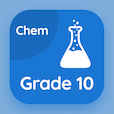SAT Online Courses

SAT Physics Quizzes

SAT Physics Quiz PDF - Complete

# SAT Physics: Radiations Quiz Questions Online p. 49

Learn SAT Physics Radiations quiz questions and answers, sat physics radiations MCQ with answers PDF 49 to learn SAT Physics online course. Transfer of Thermal Energy trivia questions, SAT Physics Radiations Multiple Choice Questions (MCQ) for online college degrees. "SAT Physics: Radiations Quiz" PDF Book: total internal reflection, energy in physics, measurement of length, friction and its effects, sat physics: radiations test prep to learn free online courses.

"The higher the temperature of the surface of the object relative to the surrounding temperature, the rate of infrared radiation will be" MCQ PDF: equal, zero, higher, and lesser for graduate school interview questions. Study transfer of thermal energy questions and answers to improve problem solving skills for high school entrance exam.

## Quiz on SAT Physics: Radiations MCQs

MCQ: The higher the temperature of the surface of the object relative to the surrounding temperature, the rate of infrared radiation will be

zero
equal
higher
lesser

MCQ: A aircraft starts from rest and its propeller produces a forward force of 30000 N. The mass of the aircraft is 35000 kg. If there is no friction then the initial acceleration of the aircraft is

1.16 ms-2
1.33 ms-2
0.85 ms-2
2.36 ms-2

MCQ: For meter rules, the zero mark is often at the rule's

end
middle
start
can be at middle or start

MCQ: The ratio for the efficiency of a machine is

(useful energy output + energy input) × 100&percent;
(useful energy output - energy input) × 100&percent;
(useful energy input / energy output × 100&percent;
(useful energy output / energy input) × 100&percent;

MCQ: A medical device using optical fibers to enable doctors to see organs inside a human body, such as intestine, is called

lens
microscope
endoscope
light pipe

### More Quizzes from SAT Physics Course